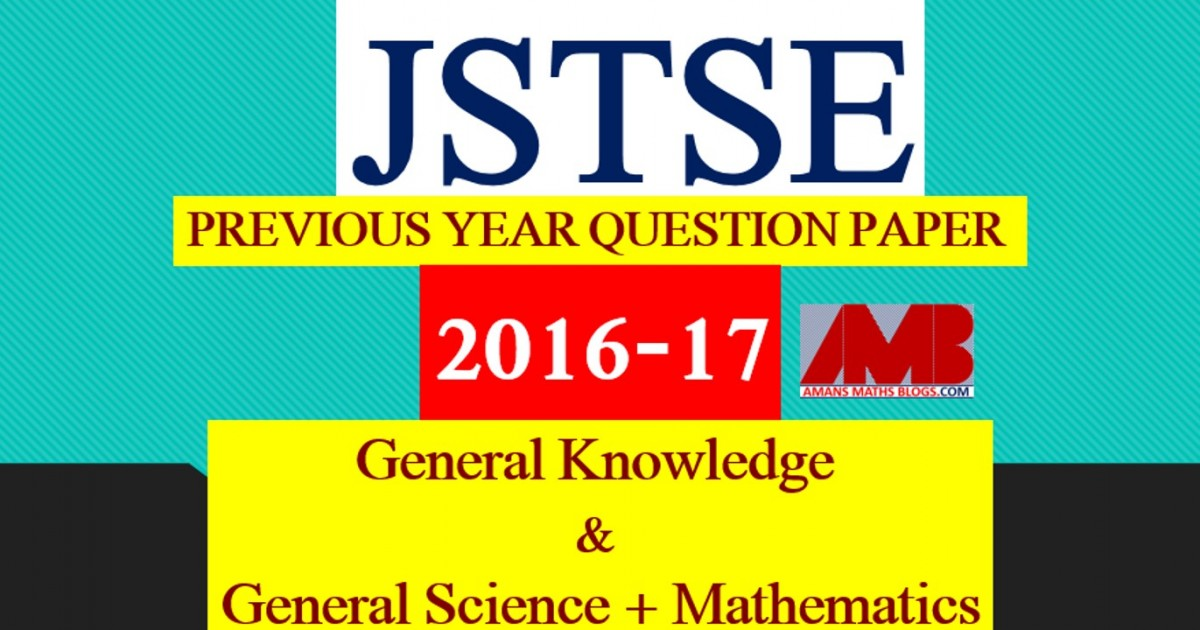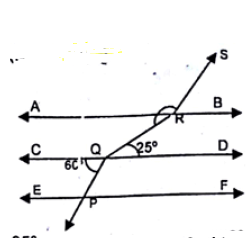Thursday, August 6, 2020
Home > download jstse papers > JSTSE PREVIOUS YEAR QUESTION PAPER 2016-17

# JSTSE PREVIOUS YEAR QUESTION PAPER 2016-17# JSTSE PREVIOUS YEAR QUESTION PAPER 2016-17

Junior Science Talent Search Examination (JSTSE) is conducted by Science Branch of DTE of Education, Delhi every year usually in the month of January for the students of recognized schools of Delhi. In this post, you will get JSTSE Previous Year Question Paper 2016-17.

JSTSE
Previous Year Question Papers
2007-082008-092009-102010-112011-12
2012-132013-142014-152015-162016-17
2018-19PhysicsChemistryMathsBiology

In JSTSE, there are two papers: Paper 1 : General Knowledge and Paper 2 :  General Science & Mathematics.

The eligibility of JSTSE exam is any students of recognizing school studying in Class 9th in Govt/Public/KV/Navodaya etc and must have secured 65% marks in Class 8th. In JSTSE, there are 150 scholarships for JSTSE per year.

Read More : 129 Maths Short Tricks

## General Science + Mathematics

JSTSE Previous Year Question Paper 2016-17 Ques No 1:

If abc = 1, then the value of (1 + a + 1/b)-1 + (1 + b + 1/c)-1 + (1 + c + 1/a)-1 is

Options:

A. 2

B. 1

C. 4

D. 8

JSTSE Previous Year Question Papers 2016-17 Ques No 2:

The value of 1/(√2 + 1) + 1/(√3 + √2) + 1/(√4 + √3) + … + 1/(√9 + √8) is

Options:

A. 4

B. 1

C. 2

D. 3

JSTSE Previous Year Question Paper 2016-17 Ques No 3:

If √(13 – a√10) = √8 + √5, then the value of a is

Options:

A. -4

B. 4

C. 2

D. -2

JSTSE Previous Year Question Papers 2016-17 Ques No 4:

It is given that ∆ABC ≅ ∆FDE and AB = 5 cm, ∠B = 40 degree and ∠A = 80 degree, then which of the following is true?

Options:

A. DF = 5 cm, ∠F = 60 degree

B. DF = 5 cm, ∠E = 60 degree

C. DE = 5 cm, ∠E = 60 degree

D. DE = 5 cm, ∠D = 40 degree

JSTSE Previous Year Question Paper 2016-17 Ques No 5:

If (a3 + 3ab2)/(3a2b + b3) = (x3 + 3xy2)/(3x2y + y3), then

Options:

A. ax = by

B. xy = ab

C. ay = bx

D. ax = b

JSTSE Previous Year Question Papers 2016-17 Ques No 6:

How much pure alcohol should be added to 400 ml of a 15% solution to make its strength 32% ?

Options:

A. 80 ml

B. 90 ml

C. 95 ml

D. 100 ml

JSTSE Previous Year Question Paper 2016-17 Ques No 7:

Simplified form of [2n+4 – 2(2n)] / [2(2n+3)] is

Options:

A. 1/8

B. 5/8

C. 7/8

D. 9/8

JSTSE Previous Year Question Papers 2016-17 Ques No 8:

If ax = cy = b and cy = az = d, then

Options:

A. xy = qz

B. x/y = q/z

C. x + y = q + z

D. x – y = q – z

JSTSE Previous Year Question Paper 2016-17 Ques No 9:

If a + b + c = 0, then the value of a2(b + c) + b2(c + a) + c2(a + b) is

Options:

A. abc

B. 3abc

C. -3abc

D. 0

JSTSE Previous Year Question Papers 2016-17 Ques No 10:

A boy is running at a speed of p km/h to cover a distance of 1 km. But due to slippery ground, his speed is reduced by q km/h. If he takes r hours to cover the same distance, then

Options:

A. 1/ r = pq/(p + q)

B. 1/ r = (p + q)

C. 1/ r = (p – q)

D. r = p – q

JSTSE Previous Year Question Paper 2016-17 Ques No 11:

If 22x-1 + 4x = 2x-1/2 + 2x+1/2, then the value of x is

Options:

A. 1/2

B. 2/3

C. 1

D. 3/2

JSTSE Previous Year Question Papers 2016-17 Ques No 12:

The price of an article was increased by p%. Later the new price was decreased by p%. If the last price was Re. 1, then original price was

Options:

A. (1 – p2)/200

B. √(1 – p2)/100

C. 1 – p2/(10000 – p2)

D. 10000/(10000 – p2)

JSTSE Previous Year Question Paper 2016-17 Ques No 13:

If x2 – 8x – 1 = 0, then x2 + 1/x2 is equal to

Options:

A. 66 + 4√3

B. 66

C. 32

D. 64

JSTSE Previous Year Question Papers 2016-17 Ques No 14:

The surface area of a sphere and a cube are equal. Then the volumes are in the ratio is

Options:

A. 6 : √π

B. √6 : √π

C. 6 : π

D. √6 : π

JSTSE Previous Year Question Paper 2016-17 Ques No 15:

If m/n = 4/3 and r/t = 9/14, then the value of (3mr – nt)/(4nr – 7mr) is

Options:

A. -5 1/2

B. -11/14

C. -1 1/4

D. 11/14

JSTSE Previous Year Question Papers 2016-17 Ques No 16:

A man buys some pens at the rate of Rs. 10 for 3 and twice these pens at the rate of Rs. 13 for 4. He sells all of them at the Rs. 59 a dozen. His profit percentage is

Options:

A. 16.67

B. 50

C. 33.33

D. 59

JSTSE Previous Year Question Paper 2016-17 Ques No 17:

A shopkeeper sells 10 kg of sugar at Rs.150. A customer asks for 15% discount and shopkeeper agrees to his demand, but instead of 1 kg, he gives only 960 gm. The approximate effective discount that the customer gets is

Options:

A. 5.5%

B. 11.46%

C. 12.64%

D. 10.5%

JSTSE Previous Year Question Papers 2016-17 Ques No 18:

The sum of the coefficient of x2 and x in the product of (x – 3)(x + 7)(x – 4) is

Options:

A. 0

B. 37

C. 84

D. -37

JSTSE Previous Year Question Paper 2016-17 Ques No 19:

If √3 = 1.732 and √2 = 1.414, then the value of 4/(3√3 – 2√2) + 3/(3√3 + 2√2)

Options:

A. 2.036

B. 2.063

C. 2.603

D. 2.306

JSTSE Previous Year Question Papers 2016-17 Ques No 20:

In the given figure, AB || CD || EF, PQ || RS, ∠RQD = 25 degree, ∠CQP = 60 degree, then ∠QRS isOptions:

A. 135 degree

B. 110 degree

C. 143 degree

D. 95 degree

JSTSE Previous Year Question Paper 2016-17 Ques No 21:

The cost price of desk and a chair is Rs. 371. If the desk costs 12% more than the chair, then the cost price of the desk is

Options:

A. Rs. 175

B. Rs. 196

C. Rs. 180

D. Rs. 200

JSTSE Previous Year Question Papers 2016-17 Ques No 22:

In the figure, X and Y are midpoints of AB and AC respectively and BC = 6 cm, AB = 5.4 cm, AC = 5 cm. Then the perimeter of the trapezium XYCB isOptions:

A. 16.4 cm

B. 14 cm

C. 14.2 cm

D. 13.4 cm

JSTSE Previous Year Question Paper 2016-17 Ques No 23:

A heptagon has 4 equal angles each of 132 degree and remaining three angles are also equal. The measure of one of the three equal angles is

Options:

A. 132 degree

B. 124 degree

C. 142 degree

D. 123 degree

JSTSE Previous Year Question Papers 2016-17 Ques No 24:

In the given figure, the value of x isOptions:

A. a + b – c

B. a – b + c

C. a + b + c

D. a – b – c

JSTSE Previous Year Question Paper 2016-17 Ques No 25:

The value of ∛(20 + 14√2) + ∛(20 – 14√2) is

Options:

A. 20

B. 14

C. 6

D. 4

JSTSE Previous Year Question Papers 2016-17 Ques No 26:

If ax + by = 3, bx – ay = 4, x2 + y2 = 1, then the value of a2 + b2 is

Options:

A. 25

B. -25

C. 16

D. -16

JSTSE Previous Year Question Paper 2016-17 Ques No 27:

If x + 1/x = 5, then the value of (x4 + 3x3 + 5x2 + 3x + 1)/(x4 + 1) is

Options:

A. 41/23

B. 43/23

C. 47/21

D. 45/21

JSTSE Previous Year Question Papers 2016-17 Ques No 28:

If x = 2√6/(√3 + √2), then the value of (x + √2)/(x – √2) + (x + √3)/(x – √3) is

Options:

A. 0

B. 1

C. 2

D. √6

JSTSE Previous Year Question Paper 2016-17 Ques No 29:

The area of a circular ring enclosed between two concentric circles is 286 cm2. If the difference between their radii is 7 cm, then the radii of these circles are

Options:

A. 12 cm and 5 cm

B. 9 cm and 2 cm

C. 11 cm and 4 cm

D. 10 cm and 3 cm

JSTSE Previous Year Question Papers 2016-17 Ques No 30:

If x = (27)1/3 and y = (28)1/3, then the value of (x + y) – 1/(x2 + xy + y2) is

Options:

A. 1

B. 3

C. 6

D. 1/3

JSTSE
Previous Year Question Papers
2007-082008-092009-102010-112011-12
2012-132013-142014-152015-162016-17
2018-19PhysicsChemistryMathsBiology
error: Content is protected !!